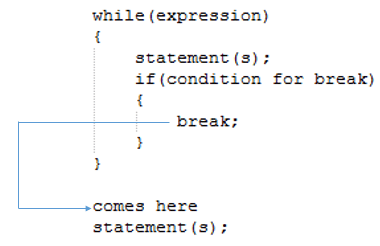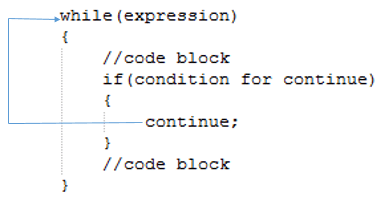# break and continue statement

It is sometimes desirable to skip some statements inside the loop or terminate the loop immediately without checking the test expression. In such cases we can use break statements in Java. Please note that Java does not provide Go To statement like other programming languages e.g. C, C++ (The Java keyword list specifies the goto keyword, but it is marked as "not used").

## Break statement

The break statement is used with the conditional switch statement and with the do, for, and while loop statements. When a break statement is encountered inside a loop, the loop is immediately terminated and the program control resumes at the next statement following the loop. Syntax:
break;As seen in the above image, the break statement ends execution of the nearest enclosing loop or conditional statement in which it appears. Control passes to the statement that follows the end of the statement, if any.

Example:
class TestClass { public static void main (String[] args) { int cnt = 1; while (cnt < = 5) { if (cnt==3) break; System.out.println("The value of cnt is : " + cnt); cnt = cnt + 1; } System.out.println("The value of cnt is : " + cnt); } }
Output:
The value of cnt is : 1 The value of cnt is : 2 The value of cnt is : 3

In the above program, when cnt==3, the break statement executed and immediately terminated the while loop and the program control resumes at the next statement.

## break statement in for loop

class TestClass { public static void main (String[] args) { int cnt; for (cnt = 1; cnt < = 5; cnt++) { if(cnt==2) break; System.out.println("Current value of cnt is: " + cnt); } System.out.println("Current value of cnt is: " + cnt); } }
Output:
Current value of cnt is: 1 Current value of cnt is: 2

## Continue statement

Continue statement works like break but instead of forcing termination, it forces the next iteration of the loop to take place and skipping the rest of the code. Continue statement is mostly used inside loops, whenever it is encountered inside a loop, either conditionally or unconditionally, transfers control to the next iteration of either the current loop or an enclosing labelled loop. Syntax:
contine;As seen in the above image, the continue statement ends current flow of execution and control directly jumps to the beginning of the loop for next iteration.

Example:
class TestClass { public static void main (String[] args) { int cnt = 0; while (cnt < 5){ cnt = cnt + 1; if (cnt==3) continue; System.out.println("The value of cnt is : " + cnt); } } }
Output:
The value of cnt is : 1 The value of cnt is : 2 The value of cnt is : 4 The value of cnt is : 5

In the baove program, we can see in the output the 3 is missing. It is because when cnt==3 the loop encounter the continue statement and control go back to start of the loop.

## continue statement in for loop

class TestClass { public static void main (String[] args) { int cnt; for (cnt = 1; cnt < 5; cnt++) { if(cnt==3) continue; System.out.println("Current value of cnt is: " + cnt); } System.out.println("Current value of cnt is: " + cnt); } }
Output:
Current value of cnt is: 1 Current value of cnt is: 2 Current value of cnt is: 4 Current value of cnt is: 5